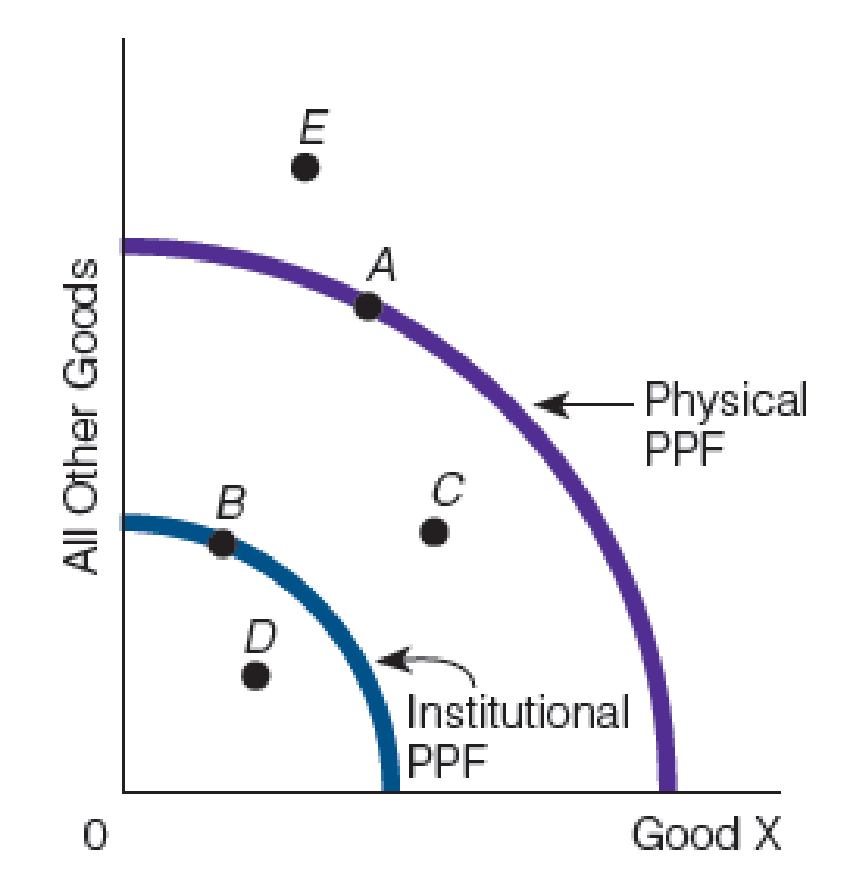Chapter 9, Problem 1WNG

Chapter
Section
Textbook Problem

In the following figure, which point is representative of a. the economy on its LRAS curve? b. the economy in a recessionary gap? c. the economy in an inflationary gap?(a)

To determine

Estimate the diagrammatic explanation for the long-run equilibrium.

Explanation

Figure-1 shows the physical PPF and institutional PPF as follows:

The long-run equilibrium refers to th...

(b)

To determine

Estimate the diagrammatic explanation for a recessionary gap.

(c)

To determine

Estimate the diagrammatic explanation for an inflationary gap.

Still sussing out bartleby?

Check out a sample textbook solution.

See a sample solution

The Solution to Your Study Problems

Bartleby provides explanations to thousands of textbook problems written by our experts, many with advanced degrees!

Get Started

Find more solutions based on key concepts